####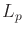metrics

The most important family of metrics over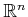is given for any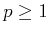as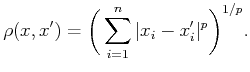(5.1)

For each value of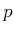, (5.1) is called anmetric (pronounced el pee''). The three most common cases are
1.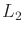: The Euclidean metric, which is the familiar Euclidean distance in.
2.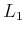: The Manhattan metric, which is often nicknamed this way because in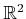it corresponds to the length of a path that is obtained by moving along an axis-aligned grid. For example, the distance from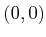to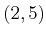is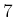by traveling east two blocks'' and then north five blocks''.
3.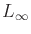: Themetric must actually be defined by taking the limit of (5.1) astends to infinity. The result is(5.2)

which seems correct because the larger the value of, the more the largest term of the sum in (5.1) dominates.
Anmetric can be derived from a norm on a vector space. Annorm overis defined as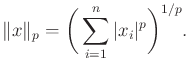(5.3)

The case of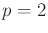is the familiar definition of the magnitude of a vector, which is called the Euclidean norm. For example, assume the vector space is, and let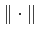be the standard Euclidean norm. Themetric is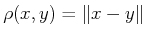. Anymetric can be written in terms of a vector subtraction, which is notationally convenient.

Steven M LaValle 2020-08-14